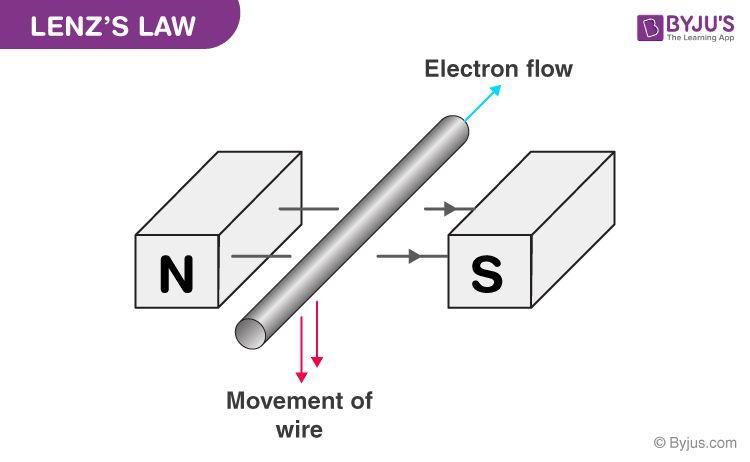# Lenz's Law

## What is Lenz’s Law?

Lenz’s law states that

The induced electromotive force with different polarities induces a current whose magnetic field opposes the change in magnetic flux through the loop in order to ensure that original flux is maintained through the loop when current flows in it.

Named after Emil Lenz, Lenz’s law depends on the principle of conservation of energy and Newton’s third law. It is the most convenient method to determine the direction of the induced current. It states that the direction of an induced current is always such as to oppose the change in the circuit or the magnetic field that produces it.

## Lenz’s Law Formula

The Lenz’s Law is reflected in the formula of Faraday’s law. Here the negative sign is contributed from Lenz’s law. The expression is –

$Emf=-N\left ( \frac{\Delta \phi }{\Delta t} \right )$

Where,

Emf is the induced voltage (also known as electromotive force).

N is the number of loops.

$\Delta \phi$ Change in magnetic flux.

$\Delta t$ Change in time.

Following is the table with links of other Physics related laws:

## Lenz’s Law Applications

Lenz’s law applications are plenty. Some of them are listed below-

• Eddy current balances
• Metal detectors
• Eddy current dynamometers
• Braking systems on train
• AC generators
• Microphones

## Lenz’s Law Experiment

To find the direction of the induced electromotive force and current we look to Lenz’s law. Some experiments were proved by Lenz’s in accordance with his theory.### First Experiment

In the first experiment, he concluded that when the current in the coil flows in the circuit the magnetic field lines are produced. As the current flows through the coil increases, the magnetic flux will increase. The direction of the flow of induced current would be such that it opposes when the magnetic flux increases.

### Second Experiment

In the second experiment, he concluded that when the current-carrying coil is wound on an iron rod with its left end behaving as N-pole and is moved towards the coil S, an induced current will be produced.

### Third Experiment

In the third experiment, he concluded that when the coil is pulled towards the magnetic flux, the coil linked with it goes on decreasing which means that the area of the coil inside the magnetic field decreases. According to Lenz’s law, the motion of the coil is opposed when the induced current is applied in the same direction.

To produce the current force is exerted by the magnet in the loop. To oppose the change a force must be exerted by the current on the magnet.

Stay tuned with BYJU’S for more such interesting articles. Also, register to “BYJU’S-The Learning App” for loads of interactive, engaging physics-related videos and an unlimited academic assist.

## Frequently Asked Questions – FAQ’s

### How is Lenz’s law conservation of energy?

Lenz’s law is based on the law of conservation of energy. From the definition of Lenz’s law, we know that the induced current is always opposed by the cause that produces it. Therefore, there is extra work done against the opposing force. The work done against the opposing force results in the change in the magnetic flux and hence the current is induced. The extra work done is known as electrical energy which is the law of conservation of energy.

### What is the difference between Lenz’s law and Faraday’s law?

Lenz’s law is about conservation of energy applied to the electromagnetic induction whereas Faraday’s law is about the electromagnetic force produced.

### What is the prime importance of Lenz’s law?

Lenz’s law is used to determine the direction of the induced current.